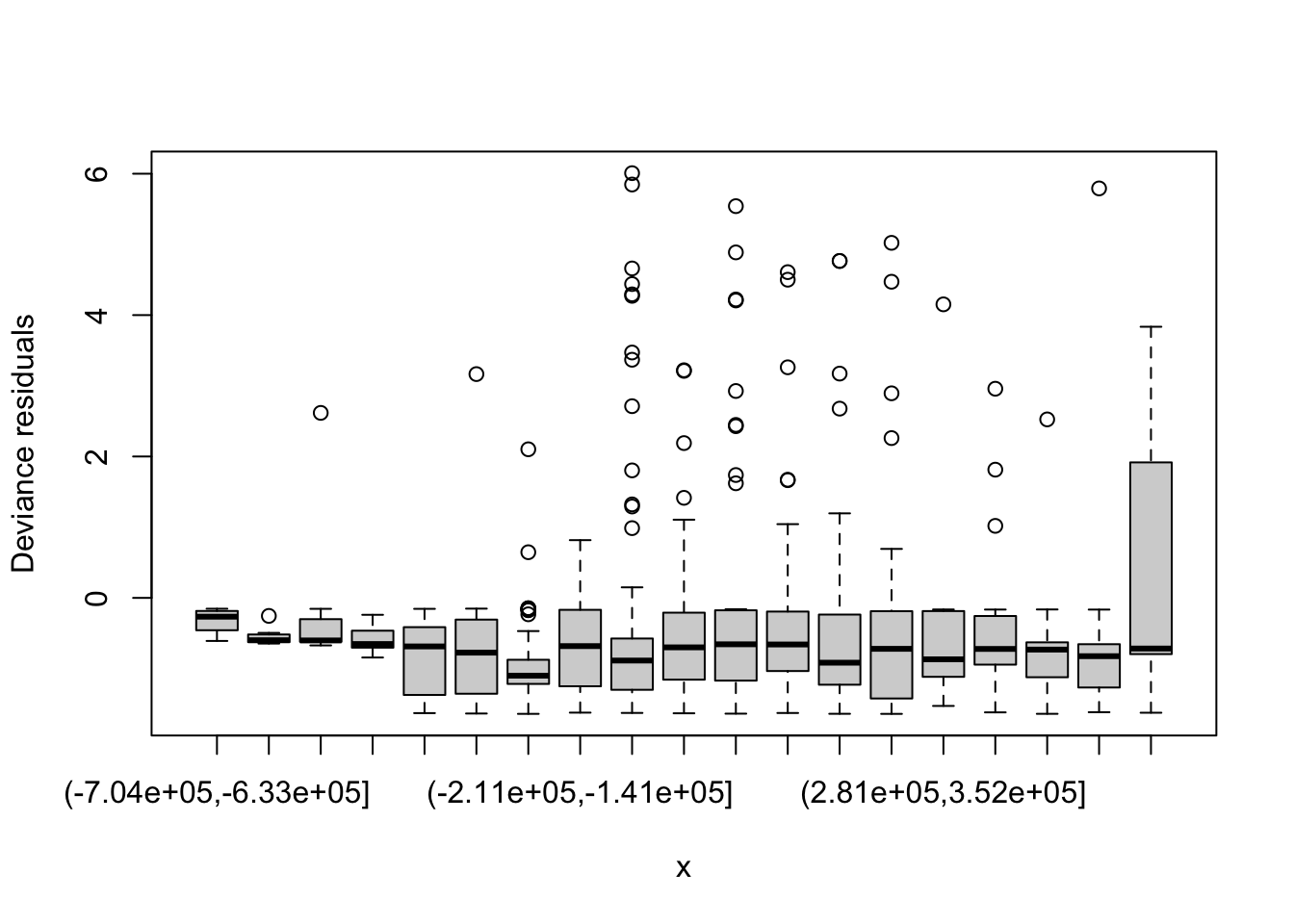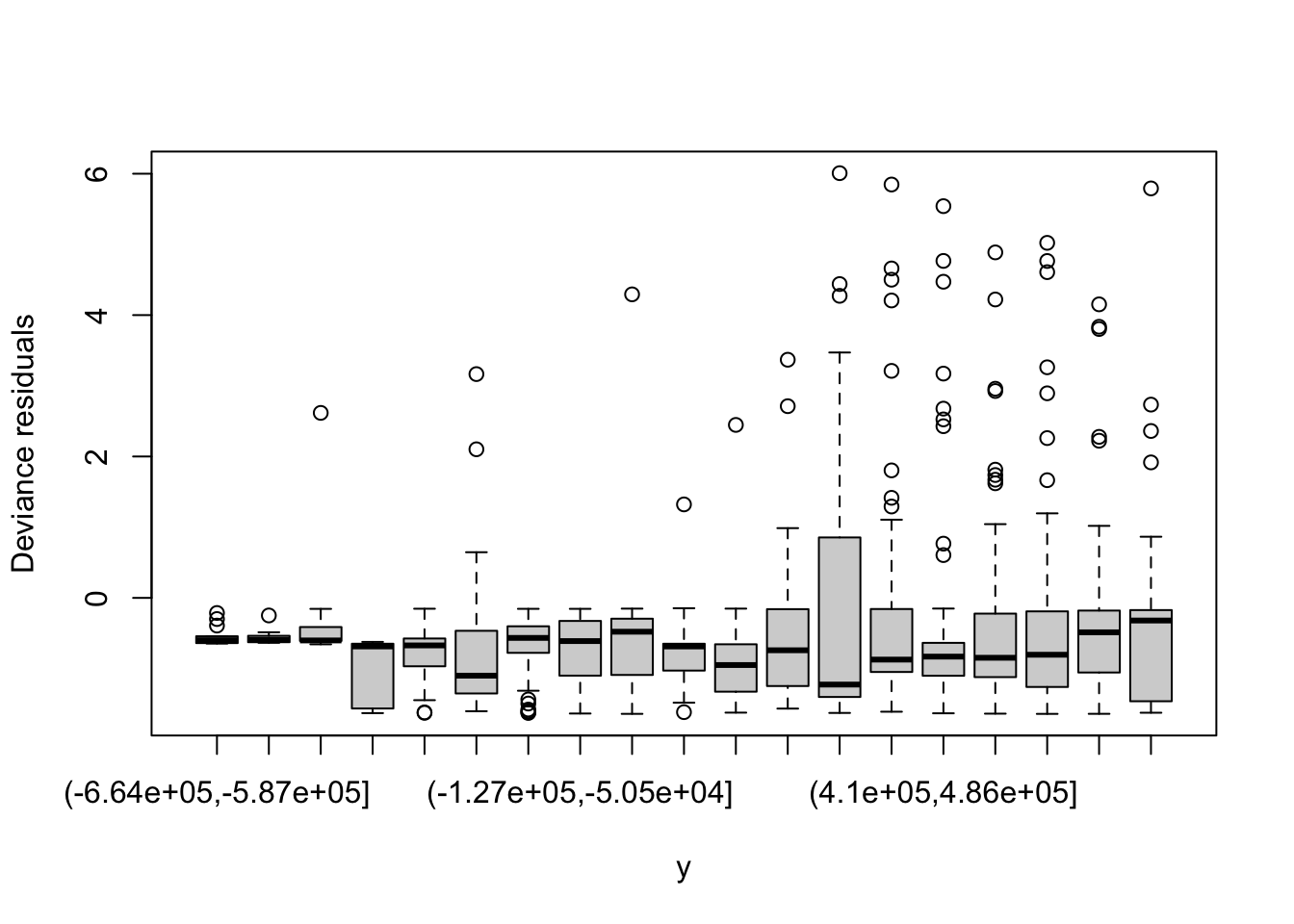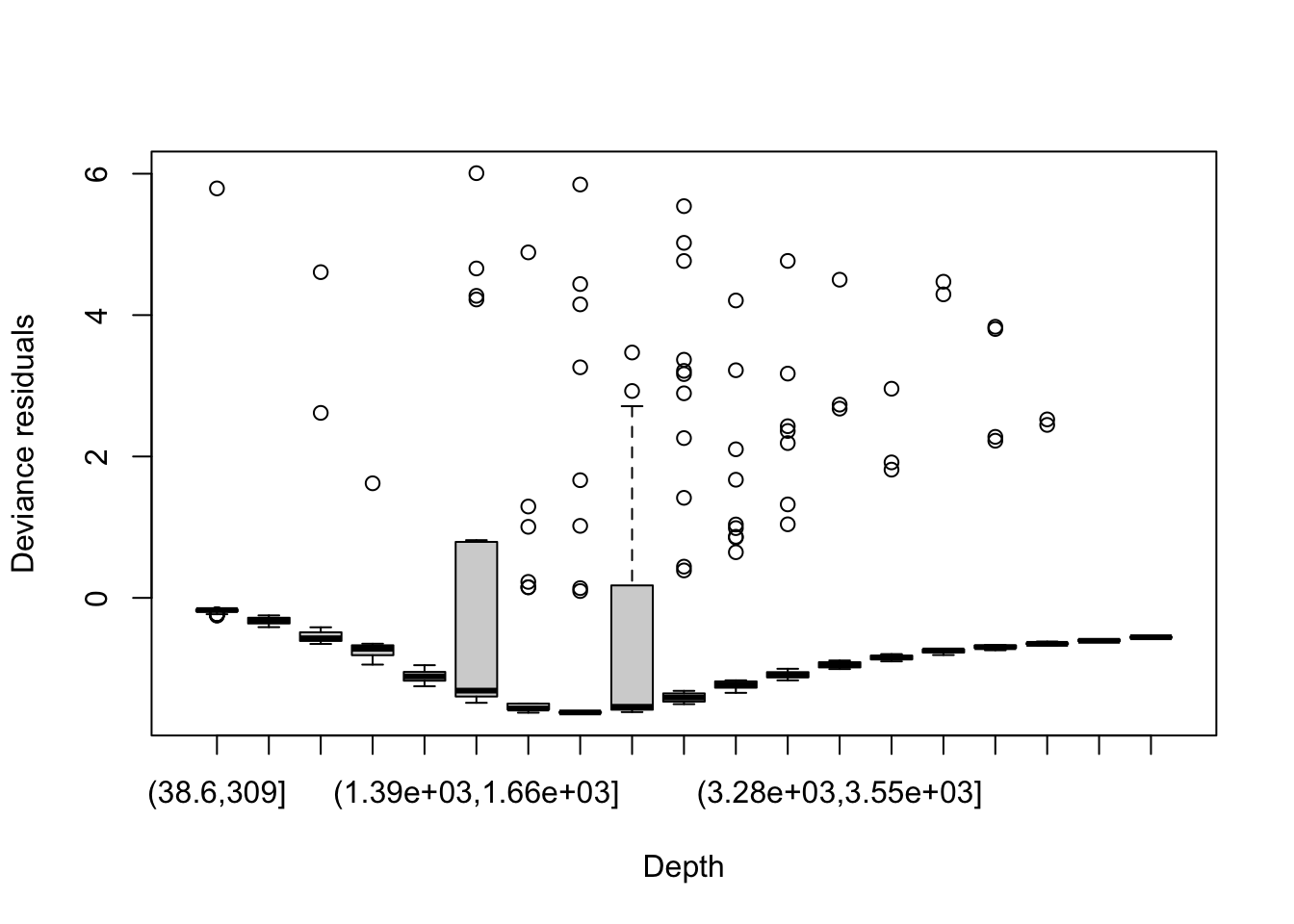## Aims

By the end of this practical, you should feel comfortable:

• Looking at how changing k changes smooths
• Plotting residuals vs. covariates
• Fitting models to the residuals
• Using gam.check and rqgam.check
• Using obs_exp

library(Distance)
## Loading required package: mrds
## This is mrds 2.2.3
## Built: R 4.0.2; ; 2020-08-01 10:33:56 UTC; unix
##
## Attaching package: 'Distance'
## The following object is masked from 'package:mrds':
##
##     create.bins
library(dsm)
## Loading required package: mgcv
## Loading required package: nlme
## This is mgcv 1.8-33. For overview type 'help("mgcv-package")'.
## Loading required package: numDeriv
## This is dsm 2.3.0
## Built: R 4.0.2; ; 2020-07-16 23:56:50 UTC; unix
library(ggplot2)
library(knitr)

Load the data and the fitted dsm objects from the previous exercises:

load("spermwhale.RData")
load("df-models.RData")

We’re going to check the DSMs that we fitted in the previous practical, then save those that we think are good!

Pre-model fitting

As we did in the previous exercise, we must remove the observations from the spatial data that we excluded when we fitted the detection function, i.e. those observations at distances greater than the truncation.

obs <- obs[obs$distance <= df_hn$ddf$meta.data$width, ]

## Looking at how changing k changes smooths

First checking that k is big enough, we should really do this during model fitting, but we’ve separated this up for the practical exercises.

First look at the text output of gam.check, are the values of k' for your models close to the edf in the outputted table. Here’s a silly example where I’ve deliberately set k too small:

dsm_k_check_eg <- dsm(count ~ s(Depth, k=4),
df_hn, segs, obs,
family=tw())
gam.check(dsm_k_check_eg)
##
## Method: REML   Optimizer: outer newton
## full convergence after 8 iterations.
## (score 392.2823 & scale 5.216272).
## Hessian positive definite, eigenvalue range [0.8702242,302.9225].
## Model rank =  4 / 4
##
## Basis dimension (k) checking results. Low p-value (k-index<1) may
## indicate that k is too low, especially if edf is close to k'.
##
##           k' edf k-index p-value
## s(Depth) 3.0 2.9    0.78    0.17

Generally if the EDF is close to the value of k you supplied, it is worth doubling k and refitting to see what happens. You can always switch back to the smaller k if there is little difference. The ?choose.k manual page can offer some guidance.

Continuing with that example, if we double k:

dsm_k_check_eg <- dsm(count ~ s(Depth, k=8),
df_hn, segs, obs,
family=tw())
gam.check(dsm_k_check_eg)
##
## Method: REML   Optimizer: outer newton
## full convergence after 8 iterations.
## (score 392.2128 & scale 5.216315).
## Hessian positive definite, eigenvalue range [1.313321,300.6316].
## Model rank =  8 / 8
##
## Basis dimension (k) checking results. Low p-value (k-index<1) may
## indicate that k is too low, especially if edf is close to k'.
##
##            k'  edf k-index p-value
## s(Depth) 7.00 4.47    0.78    0.26

We get something much more reasonable. Doubling again

dsm_k_check_eg <- dsm(count ~ s(Depth, k=16),
df_hn, segs, obs,
family=tw())
gam.check(dsm_k_check_eg)
##
## Method: REML   Optimizer: outer newton
## full convergence after 7 iterations.
## (score 392.2614 & scale 5.213115).
## Hessian positive definite, eigenvalue range [1.440887,300.5744].
## Model rank =  16 / 16
##
## Basis dimension (k) checking results. Low p-value (k-index<1) may
## indicate that k is too low, especially if edf is close to k'.
##
##             k'   edf k-index p-value
## s(Depth) 15.00  4.83    0.78    0.17

We see almost no improvement, so might refit the second model here with k=8.

Check to see if any of your models need this treatment.

Checking k size

I saved the following models last time: dsm_nb_xy_ms_p1_4, dsm_tw_xy_ms, dsm_nb_noxy_ms_p1_3, dsm_nb_xy, dsm_tw_xy, dsm_nb_xy_ms_ae and dsm_tw_xy_ms_ae.

I’ll run gam.check on each. Note that I’ve used the fig.show='hide' “chunk” option in RMarkdown to suppress the plots here and save space

gam.check(dsm_nb_xy_ms_p1_4)
##
## Method: REML   Optimizer: outer newton
## full convergence after 6 iterations.
## (score 382.759 & scale 1).
## Hessian positive definite, eigenvalue range [0.3417744,30.6163].
## Model rank =  48 / 48
##
## Basis dimension (k) checking results. Low p-value (k-index<1) may
## indicate that k is too low, especially if edf is close to k'.
##
##              k'    edf k-index p-value
## s(x,y)   29.000  1.864    0.55  <2e-16 ***
## s(Depth)  9.000  3.418    0.69   0.045 *
## s(EKE)    9.000  0.856    0.74   0.680
## ---
## Signif. codes:  0 '***' 0.001 '**' 0.01 '*' 0.05 '.' 0.1 ' ' 1
gam.check(dsm_tw_xy_ms)
##
## Method: REML   Optimizer: outer newton
## full convergence after 11 iterations.
## (score 385.0946 & scale 4.573293).
## Hessian positive definite, eigenvalue range [0.3357644,301.6658].
## Model rank =  48 / 48
##
## Basis dimension (k) checking results. Low p-value (k-index<1) may
## indicate that k is too low, especially if edf is close to k'.
##
##              k'    edf k-index p-value
## s(x,y)   29.000  1.897    0.62  <2e-16 ***
## s(Depth)  9.000  3.695    0.81    0.48
## s(EKE)    9.000  0.811    0.82    0.52
## ---
## Signif. codes:  0 '***' 0.001 '**' 0.01 '*' 0.05 '.' 0.1 ' ' 1
gam.check(dsm_nb_noxy_ms_p1_3)
##
## Method: REML   Optimizer: outer newton
## full convergence after 6 iterations.
## (score 383.0449 & scale 1).
## Hessian positive definite, eigenvalue range [0.3565081,30.73282].
## Model rank =  28 / 28
##
## Basis dimension (k) checking results. Low p-value (k-index<1) may
## indicate that k is too low, especially if edf is close to k'.
##
##             k'   edf k-index p-value
## s(Depth) 9.000 3.415    0.69   0.025 *
## s(EKE)   9.000 0.866    0.74   0.700
## s(NPP)   9.000 2.814    0.68   0.025 *
## ---
## Signif. codes:  0 '***' 0.001 '**' 0.01 '*' 0.05 '.' 0.1 ' ' 1
gam.check(dsm_nb_xy)
##
## Method: REML   Optimizer: outer newton
## full convergence after 4 iterations.
## (score 394.963 & scale 1).
## Hessian positive definite, eigenvalue range [2.166966,29.85537].
## Model rank =  25 / 25
##
## Basis dimension (k) checking results. Low p-value (k-index<1) may
## indicate that k is too low, especially if edf is close to k'.
##
##          k'  edf k-index p-value
## s(x,y) 24.0 13.9    0.51  <2e-16 ***
## ---
## Signif. codes:  0 '***' 0.001 '**' 0.01 '*' 0.05 '.' 0.1 ' ' 1
gam.check(dsm_tw_xy)
##
## Method: REML   Optimizer: outer newton
## full convergence after 7 iterations.
## (score 396.9528 & scale 4.966045).
## Hessian positive definite, eigenvalue range [2.604446,301.2817].
## Model rank =  25 / 25
##
## Basis dimension (k) checking results. Low p-value (k-index<1) may
## indicate that k is too low, especially if edf is close to k'.
##
##          k'  edf k-index p-value
## s(x,y) 24.0 14.5     0.6  <2e-16 ***
## ---
## Signif. codes:  0 '***' 0.001 '**' 0.01 '*' 0.05 '.' 0.1 ' ' 1
gam.check(dsm_nb_xy_ms_ae)
##
## Method: REML   Optimizer: outer newton
## full convergence after 7 iterations.
## (score 505.0342 & scale 1).
## Hessian positive definite, eigenvalue range [0.2941817,44.37103].
## Model rank =  48 / 48
##
## Basis dimension (k) checking results. Low p-value (k-index<1) may
## indicate that k is too low, especially if edf is close to k'.
##
##              k'    edf k-index p-value
## s(x,y)   29.000  1.335    0.46  <2e-16 ***
## s(Depth)  9.000  3.503    0.55   0.025 *
## s(EKE)    9.000  0.772    0.60   0.795
## ---
## Signif. codes:  0 '***' 0.001 '**' 0.01 '*' 0.05 '.' 0.1 ' ' 1
gam.check(dsm_tw_xy_ms_ae)
##
## Method: REML   Optimizer: outer newton
## full convergence after 11 iterations.
## (score 455.5013 & scale 8.662789).
## Hessian positive definite, eigenvalue range [0.3430708,293.2559].
## Model rank =  48 / 48
##
## Basis dimension (k) checking results. Low p-value (k-index<1) may
## indicate that k is too low, especially if edf is close to k'.
##
##              k'    edf k-index p-value
## s(x,y)   29.000  1.900    0.66  <2e-16 ***
## s(Depth)  9.000  3.772    0.84    0.59
## s(EKE)    9.000  0.826    0.84    0.60
## ---
## Signif. codes:  0 '***' 0.001 '**' 0.01 '*' 0.05 '.' 0.1 ' ' 1

(That might have taken a while to run!)

Sifting through these results, most of the cases were the $$p$$-value is significant (at any level beyond 0.1) it’s the case that the EDF is far from $$k^\prime$$. The only models I am worried about are dsm_nb_xy and dsm_tw_xy where the $$p$$-value is singificant and $$k^\prime$$ is “near” the EDF (it’s not that near but is is over half, so let’s check it out).

For the Tweedie model we set k=25 and got an EDF of 14.5, so we’ll double k to 50:

dsm_tw_xy <- dsm(count~s(x,y, bs="ts", k=50),
df_hn, segs, obs,
family=tw())
summary(dsm_tw_xy)
##
## Family: Tweedie(p=1.283)
##
## Formula:
## count ~ s(x, y, bs = "ts", k = 50) + offset(off.set)
##
## Parametric coefficients:
##             Estimate Std. Error t value Pr(>|t|)
## (Intercept) -20.6281     0.2518  -81.92   <2e-16 ***
## ---
## Signif. codes:  0 '***' 0.001 '**' 0.01 '*' 0.05 '.' 0.1 ' ' 1
##
## Approximate significance of smooth terms:
##          edf Ref.df     F p-value
## s(x,y) 20.34     49 2.068  <2e-16 ***
## ---
## Signif. codes:  0 '***' 0.001 '**' 0.01 '*' 0.05 '.' 0.1 ' ' 1
##
## R-sq.(adj) =  0.125   Deviance explained = 37.5%
## -REML = 406.92  Scale est. = 4.6374    n = 949
gam.check(dsm_tw_xy)
##
## Method: REML   Optimizer: outer newton
## full convergence after 6 iterations.
## (score 406.9169 & scale 4.637444).
## Hessian positive definite, eigenvalue range [1.816522,297.6612].
## Model rank =  50 / 50
##
## Basis dimension (k) checking results. Low p-value (k-index<1) may
## indicate that k is too low, especially if edf is close to k'.
##
##          k'  edf k-index p-value
## s(x,y) 49.0 20.3    0.62  <2e-16 ***
## ---
## Signif. codes:  0 '***' 0.001 '**' 0.01 '*' 0.05 '.' 0.1 ' ' 1

Okay that’s a moderate jump there! We can see now the EDF is less than half of $$k^\prime$$ but as we can see the $$p$$-value is still significant. It’s not likely that increasing $$k$$ will do anything here. As I said in the lecture, we need to look at $$k^\prime$$, the EDF and the $$p$$-value here to see what’s going on.

We can do the same thing with the negative binomial model, there the EDF was 13.9 when k=25.

dsm_nb_xy <- dsm(count~s(x,y, bs="ts", k=50),
df_hn, segs, obs,
family=nb())
summary(dsm_nb_xy)
##
## Family: Negative Binomial(0.108)
##
## Formula:
## count ~ s(x, y, bs = "ts", k = 50) + offset(off.set)
##
## Parametric coefficients:
##             Estimate Std. Error z value Pr(>|z|)
## (Intercept) -20.6787     0.2423  -85.35   <2e-16 ***
## ---
## Signif. codes:  0 '***' 0.001 '**' 0.01 '*' 0.05 '.' 0.1 ' ' 1
##
## Approximate significance of smooth terms:
##          edf Ref.df Chi.sq p-value
## s(x,y) 19.75     49  81.49  <2e-16 ***
## ---
## Signif. codes:  0 '***' 0.001 '**' 0.01 '*' 0.05 '.' 0.1 ' ' 1
##
## R-sq.(adj) =  0.102   Deviance explained = 41.6%
## -REML = 405.47  Scale est. = 1         n = 949
gam.check(dsm_nb_xy)
##
## Method: REML   Optimizer: outer newton
## full convergence after 4 iterations.
## (score 405.4683 & scale 1).
## Hessian positive definite, eigenvalue range [1.705091,27.92868].
## Model rank =  50 / 50
##
## Basis dimension (k) checking results. Low p-value (k-index<1) may
## indicate that k is too low, especially if edf is close to k'.
##
##          k'  edf k-index p-value
## s(x,y) 49.0 19.8    0.54  <2e-16 ***
## ---
## Signif. codes:  0 '***' 0.001 '**' 0.01 '*' 0.05 '.' 0.1 ' ' 1

Again we see a wee jump in the EDF but the $$p$$-value is still significant. For the same reasons as above, we’re not going to worry too much about that.

## Plotting residuals vs. covariates

Now following from the slides, let’s try plotting residuals vs. covariates. We can use these diagnostics (along with those in the following section) to assess how well the model has accounted for the structure in the data. This involves a little data manipulation and a little thought about how to chop up the covariate values.

I’ll show an example here using the not very good model we fitted above, so we can see what happens when things go wrong:

dsm_k_low <- dsm(count ~ s(Depth, k=4),
df_hn, segs, obs,
family=tw())

# get the data used to fit the model
resid_dat <- dsm_k_low$data # get the residuals resid_dat$resids <- residuals(dsm_k_low)

# now let's chop x into 20 chunks
resid_dat$x_chop <- cut(resid_dat$x,
seq(min(resid_dat$x), max(resid_dat$x), len=20))

# and plot the boxplot
boxplot(resids~x_chop, data=resid_dat, ylab="Deviance residuals", xlab="x")This seems to show that something is going on in the far east of the data – there’s a wide range of residuals there. Note that for our data the most easterly points are also North (since our data is somewhat diagonal in shape, with respect to the compass). So let’s see if this is an issue with x alone (East-West) or y (North-Southness) is an issue too.

# now let's chop y into 20 chunks
resid_dat$y_chop <- cut(resid_dat$y,
seq(min(resid_dat$y), max(resid_dat$y), len=20))

# and plot the boxplot
boxplot(resids~y_chop, data=resid_dat, ylab="Deviance residuals", xlab="y")We can also do this chopping with Depth (the only covariate in this model):

# now let's chop Depth into 20 chunks
resid_dat$Depth_chop <- cut(resid_dat$Depth,
seq(min(resid_dat$Depth), max(resid_dat$Depth), len=20))

boxplot(resids~Depth_chop, data=resid_dat, ylab="Deviance residuals", xlab="Depth")Now we see something more pathological, there is pattern in these residuals.

Let’s make the same plot for a model with an spatial smooth in it:

dsm_better <- dsm(count ~ s(x,y) + s(Depth),
df_hn, segs, obs,
family=tw())

# get the data from the larger k model
resid_dat <- dsm_better$data # get the residuals resid_dat$resids <- residuals(dsm_better)

# now let's chop Depth into 20 chunks
resid_dat$Depth_chop <- cut(resid_dat$Depth,
seq(min(resid_dat$Depth), max(resid_dat$Depth), len=20))

boxplot(resids~Depth_chop, data=resid_dat, ylab="Deviance residuals", xlab="Depth")